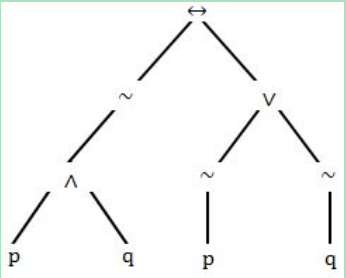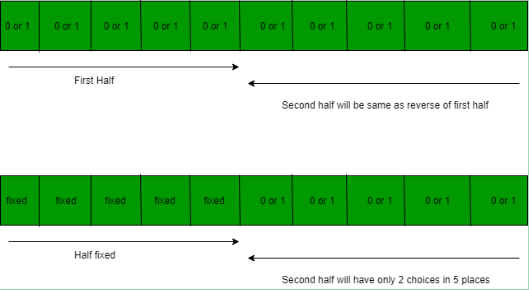# UGC NET COMPUTER SCIENCE SOLVED PAPERS 2014-16 - UGC NET Computer Science Paper 2 August 2016

>>>>>>>>UGC NET Computer Science Paper 2 August 2016

• A

q• B

p ∧ r• C

p ∨ q• D

p• Option : D
• Explanation :
We have Boolean function: [~(~p ∧ q) ∧ ~( ~p ∧ ~q)] ∨ (p ∧ r)] = [(p ∨ ~q) ∧ (p ∨ q ) ∨ (p ∧ r)] = [p ∨ (p ∧ q) ∨ (p ∧ ~q) ∨(p ∧ r)] = p[1 ∨ q ∨ ~q ∨ r] = p So, option (D) is correct.

• A

↔~∧pq∨ ~ ~ pq, pq∧~p~q~∨↔• B

↔~∧pq∨ ~ p~q, pq∧~p~q~∨↔• C

↔~∧pq∨ ~ ~ pq, pq∧~p~~q∨↔• D

↔~∧pq∨ ~ p~ q, pq∧~p~ ~q∨↔• Option : B
• Explanation :
We have compound proposition (~ (p ∧ q)) ↔ (~ p ∨ ~ q): Now we will construct ordered tree:We are asked to determine pre-order (i.e. parent-node left-node right-node), we will drive it from ordered tree i.e. ↔ ~ ∧ p q ∨ ~ p ~q And post-order(i.e. left-node right-node parent-node) from the ordered tree it is p q ∧ ~ p ~ q ~ ∨ ↔. So, option (B) is correct.

• A

|A – B| < |A ⊕ B| < |A| + |B| < |A ∪ B|• B

|A ⊕ B| < |A – B| < |A ∪ B| < |A| + |B|• C

|A ⊕ B| < |A| + |B| < |A – B| < |A ∪ B|• D

|A – B| < |A ⊕ B| < |A ∪ B| < |A| + |B|• Option : D
• Explanation :
We will draw venn diagram for all set: |A – B|, |A ⊕ B|, |A| + |B| and |A ∪ B|So, option (D) is correct. Alternative way -
|A – B| = |A| - |A ∩ B|

|A ⊕ B| = |A| + |B| - 2|A ∩ B|
|A ∪ B| = |A| + |B| - |A ∩ B|
Therefore,
|A – B| < |A ⊕ B| < |A ∪ B| < |A| + |B|

• A

1/64• B

1/32• C

1/8• D

1/4• Option : B
• Explanation :
In the given question we have a palindrome: in even length palindrome half length is fixed and rest is repeated. So, in 10 bit palindrome, we have 5 position to be filled with 2 choices each-i.e. 25 choices for first half and 25 choices for second half.
Probability = favorable outcome / total outcome
= 25/ 210
= 1 / 25
= 1 / 32.
So, option (B) is correct.

• Option : C
• Explanation :
Euler circuit does not contain odd length cycle. Refer: Eulerian path and circuit for undirected graph None of the above graph is Eulerian. So, option (C) is correct.